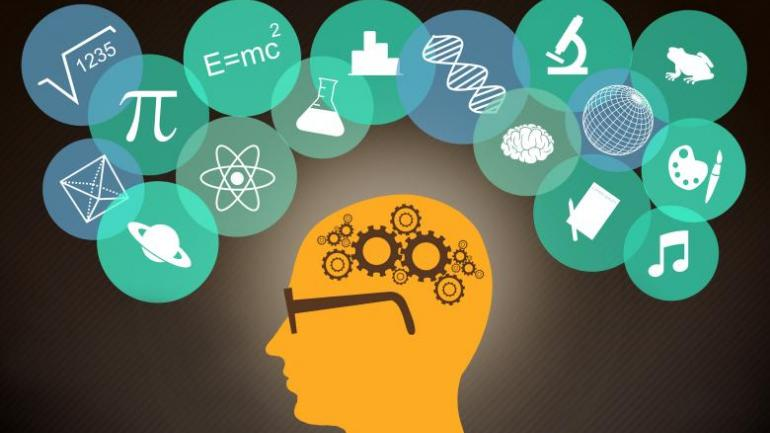## CBSE Class 12 Science: Subject-wise preparation tips for PCMThese preparation tips for CBSE Class 12 Science board exam will surely help you score good marks!

With another 4 weeks to go before the CBSE Class 12 board exams begin, students from the science stream (ie PCM group) are now gearing up for it. Most of these students would have appeared in JEE Main 2019 (in January) and now their focus is to maximize their performance in the board exam.

### Here are 7 preparation tips for your Class 12 board exam that can help:

1. Understand the syllabus and make proper study plan: By assessing the weightage attached to different sections, you can easily decide the time required to be devoted for revision. Practicing 5- or 6-mark questions will certainly help.

2. Set daily targets: Set daily study goals in concrete terms and put it in writing to strengthen your resolve.

3. Make revision notes: Make separate notebooks for theorems, formulae and methodologies. This will help to read and brush up concepts fast.

4. Practice problems yourself: Try to solve problems by yourself in the first attempt. If you are unable to do so, only then refer to the solution or discuss it with your teacher.

5. Practice solving question papers: It is a good way to mentally prepare and be exam ready. The more you practice and solve question papers the more confident you become.

7. Analyze your mistakes: It’s extremely crucial to spot and analyze mistakes if you do not want to repeat it in the actual exam.

Read: CBSE Class 12 Board examiners list silly errors in answer sheets: Subject-wise common mistakes for Science

### CBSE Class 12 Mathematics tips

Mathematics as a subject helps in developing rational and logical thinking and hence a systematic learning is essential. Following tips will help to improve your score:

1. The long answer type questions (i.e. of 6 marks) in CBSE Class 12 Mathematics will be dreadful aspects of your exam. You should start by answering these questions so that you are able to devote good quality time on them. This can also increase your confidence which will be carried through to the rest of the exam.

2. The most important topic where you can score is Calculus (it carries 44% weightage). Focus on unit of sum, maxima and minima application problems, application of integrals (do practice on plotting graphs & labelling them)

3. The topic Differential Equations is known to contain application based difficult questions.

4. The next important topic is Vectors and 3D Geometry with 17% weightage.

5. ProbabilityRelations & Functions and Algebra have weightage 10%10% & 13% respectively.

6. Linear programming has 6% weightage. A little practice can fetch you 6 marks.

7. Other topics like Matrics and DeterminantsMatrix Methodinverse of square matrix and properties of determinants are very important whereas concepts of line and plane in 3Drequires little bit of imagination skills and Bayes Theorem in probability.

Read: Class 12 Maths tips for board exam and JEE: How to study Hyperbola

### CBSE Class 12 Chemistry tips

Physical Chemistry

1. In case you have studied from the NCERT book before (may be for school exam/pre board exam) then you just need to do a quick revision of the topics and then solve the NCERT problems in the text questions and then, the exercises.

2. In case you have not studied the NCERT book before, then you must revise important topics as listed in Learning Objective’ in the beginning of each chapter and then solve NCERT problems.

3. Write the important formulae on a sheet and revise it daily.

Organic Chemistry

1. This is a scoring section but requires systematic revision. Typically, the types of questions asked are:

• Conceptual questions (reasoning based)
• Conversions/Word Problem
• Name Reactions
• Distinction Test
• Mechanism of reaction (as per NCERT)

2. A lot of practice is needed so try to solve as many questions as you can on a daily basis.

3. Don’t just read; write the solution yourself several times to develop that flow and gain speed and accuracy. Remember, balancing of reaction is not needed.

4. Most of the students ignore and/or do not revise certain chapters like PolymersBio-molecular and Chemistry in everyday life. But these chapters collectively carry a weightage of 10 marks and hence, one must devote some time to revise them.

Inorganic Chemistry

1. In this section, questions asked are:

• Reasoning-based
• Structure-based
• Complete the reaction

2. p-block elements: Write all important reactions in a sheet and revise them on a regular basis. Practice the questions to develop that flow of writing.

3. d- and f- block elements : Revise and practice reasoning based questions; revise preparation structure and properties of compounds like KMnO4 and K2Cr2O7

4. Coordination Compound: It’s a very important chapter as type of questions asked are different. So devote good amount of time for this.

5. Metallurgy: A wide variety of questions are asked from this chapter hence it needs to be revised thoroughly.

Attempt old board questions papers and attempt mock test papers: Practice by solving old board exam question papers within a fixed time limit. This will help to develop exam temperament, flow and speed and most importantly everything will get refreshed in mind. Then attempt a few mock test papers in the last few days ahead of the exam.

Read: Class 12 Chemistry tips for board exam and JEE: How to study alcohols

### CBSE Class 12 Physics tips

1. Electrostatics and Optics are quite lengthy chapters. Hence you must devote sufficient time to revise these chapters thoroughly.

2. There could be few subjective questions based on CyclotronVan-de graff and other similar apparatus. Hence practice such questions.

3. Do not neglect small chapters like Alternating Current.

4. You will have to be thorough with the following chapters:

• Magnetic properties of materials
• Semi-conductor
• Diffraction
• Polarization
• Optical Instrument
• Electromagnetic Wave and Communication System

5. Long answer type questions should have enough explanation on all the things as mentioned.

6. While solving numerical-type problems, ensure that you are putting correct values and that the calculations are correct and well described.

[“source=indiatoday”]